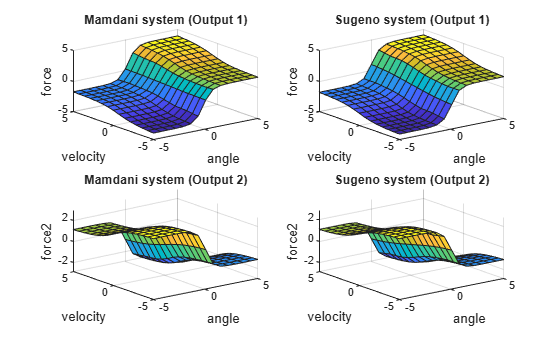# convertToSugeno

Convert Mamdani fuzzy inference system into Sugeno fuzzy inference system

## Syntax

``sugenoFIS = convertToSugeno(mamdaniFIS)``

## Description

example

````sugenoFIS = convertToSugeno(mamdaniFIS)` converts the Mamdani fuzzy inference system `mamdaniFIS` into a Sugeno fuzzy inference system `sugenoFIS`.```

## Examples

collapse all

Load a Mamdani fuzzy inference system.

`mam_fis = readfis('mam22.fis');`

Convert this system to a Sugeno fuzzy inference system.

`sug_fis = convertToSugeno(mam_fis);`

Plot the output surfaces for both fuzzy systems.

```subplot(2,2,1) gensurf(mam_fis) title('Mamdani system (Output 1)') subplot(2,2,2) gensurf(sug_fis) title('Sugeno system (Output 1)') subplot(2,2,3) gensurf(mam_fis,gensurfOptions('OutputIndex',2)) title('Mamdani system (Output 2)') subplot(2,2,4) gensurf(sug_fis,gensurfOptions('OutputIndex',2)) title('Sugeno system (Output 2)')```The output surfaces for both systems are similar.

## Input Arguments

collapse all

Mamdani fuzzy inference system, specified as a `mamfis` or `mamfistype2` object.

`mamdaniFIS` must not contain rules with NOT logic in the consequent.

## Output Arguments

collapse all

Sugeno fuzzy inference system, returned as one of the following:

`sugenoFIS`:

• Has constant output membership functions, whose values correspond to the centroids of the output membership functions in `mamdaniFIS`

• Uses the weighted-average defuzzification method

• Uses the product implication method

• Uses the sum aggregation method

The remaining properties of `sugenoFIS`, including the input membership functions and rule definitions remain unchanged from `mamdaniFIS`.

## Tips

• If you have a functioning Mamdani fuzzy inference system, consider using `convertToSugeno` to convert to a more computationally efficient Sugeno structure to improve performance.

## Alternative Functionality

### App

You can interactively convert a Mamdani FIS into a Sugeno FIS using the Fuzzy Logic Designer app. You can then export the system to the MATLAB® workspace.

## Version History

Introduced in R2018b

expand all